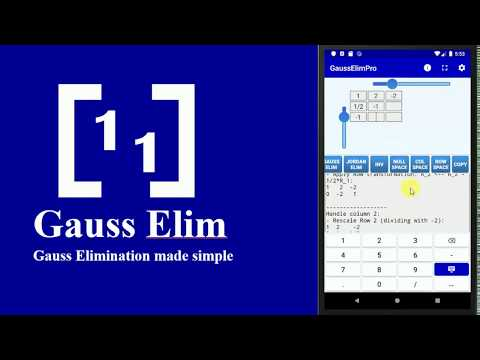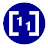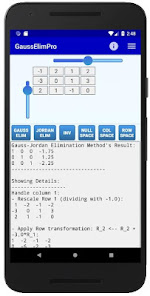# Gauss Jordan Elimination Pro100+EveryoneGauss Elim is a simple calculator that applies the Gaussian Elimination process to a given matrix. GaussElim uses fractions and makes precise calculations. You can set the matrix dimensions using the scrollbars and then you can edit the matrix elements by typing in each cell (the cells become active/inactive once you move the respective scrollbar). You can move to another cell either by pressing the NEXT key on the soft keyboard, or by tapping the desired cell.

After you have entered the entries of the desired matrix, you can press one of the available buttons and see the result (and detailed explanation) on the bottom of the screen:

Gauss Elimination Button: Applies the Gauss elimination process to the given matrix. The result is an unreduced Row-Echelon matrix.

Jordan Elimination Button: Applies the Gauss-Jordan elimination process to the given matrix. The result is a reduced Row-Echelon matrix.

INV button: Applies the Gauss-Jordan elimination process to find (if possible) the inverse of the given matrix.

Null Space button: Finds the Null space of the given matrix by applying the Gauss-Jordan Elimination Process.

Col Space button: Finds the column space of the given matrix by applying the Gauss Jordan elimination process to the transpose matrix.

Row Space button: Finds the row space of the given matrix by applying the Gauss-Jordan elimination process.
Updated on
May 28, 2020

## Data safety